# Galois field structure

(diff) ← Older revision | Latest revision (diff) | Newer revision → (diff)

Galois field (update)

This article contains some additional information concerning the structural properties of a Galois field extension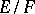, where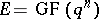and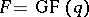; this is also of interest for computational applications. Usuallyis represented as an-dimensional vector space over, so that addition of elements ofbecomes trivial, given the arithmetics in(which, in applications, usually is a prime field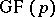represented as the residues modulo). However, the choice of a basis is crucial for performing multiplication, inversion and exponentiation. Various types of bases have been studied extensively. The most obvious choice is that of a polynomial basis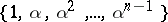, whereis a root of an irreducible polynomial of degreeover(so thatgeneratesover, cf. Galois field). In this context, one often prefersto be a generator of the cyclic group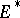(cf. Galois field); thenis usually called a primitive element or a primitive root for, and the polynomialis called a primitive polynomial. Note that these terms carry a different meaning in the context of Galois fields than in algebra in general, see Galois theory and Primitive polynomial.

The standard alternative to using a polynomial basis is a normal basis, that is, a basis of the form, cf. Normal basis theorem. Hence such a basis consists of an orbit of maximal lengthunder the Frobenius automorphism. The elementis called a free element (or a normal element) in. A stronger result is the existence of an element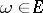that is simultaneously free in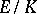for every intermediate field; such an element is called completely free (or completely normal). A constructive treatment of normal bases and completely free elements in Galois fields can be found in [a7].

Much current research (as of 2001) concerns the construction of primitive and/or free elements with additional properties. The seminal result in this direction is the primitive normal basis theorem: There always exists a primitive elementthat is simultaneously free over. This result is due to A.K. Lenstra and R.J. Schoof [a15], see also [a10]. In this context the concepts of trace and norm play an important role. For any Galois extensionwith Galois group, one defines the trace and the norm (over) of an elementas the sum and the product of all conjugates,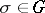, respectively (each taken with the appropriate multiplicity). In the special case under consideration, there are explicit formulas: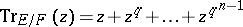(a1)

and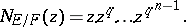(a2)

Now, letbe an irreducible polynomial over, and letbe a root of(generating). ThenThere are many results on the existence of primitive and/or (completely) free elementswith prescribed trace and/or norm, or with other prescribed coefficients. The first of these is due to S.D. Cohen [a4]: Given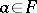, where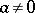if eitheror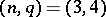, there exists a primitive elementofwith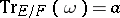. For more results of this type, see [a10].

Given any ordered basis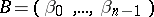of, there exists a unique dual basis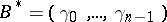, defined by the property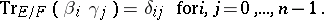One callsself-dual if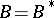. A self-dual basis forexists if eitheris even or bothandare odd. It is easily checked that the dual basis of a normal basis is likewise a normal basis; a self-dual normal basis forexists if eitheris even andis not a multiple of, or bothandare odd. The number of bases of these types has also been determined. For computational purposes (in particular, for hardware implementations), it would be desirable to have a self-dual polynomial basis; unfortunately, such bases do not exist. If one slightly weakens the requirements, a suitable substitute can be found in the so-called weakly self-dual polynomial bases; these belong to irreducible binomials and irreducible trinomials with constant term. Therefore the existence of such trinomials is an important (as of 2001 still open) question. These topics are discussed in detail in [a10] and [a12].

There is an alternative to using basis representations for finite fields: If one represents the non-zero elements of a Galois fieldas the powers of a primitive element, multiplication is trivial, but addition then becomes difficult. For any element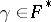, the discrete logarithm of(to the base) is the unique integerwith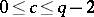satisfying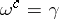; one writes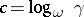and also puts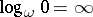. Identifying the elements ofwith their discrete logarithms, multiplying two elements reduces to adding the corresponding discrete logarithms:where the addition is done modulo. In order to perform additions in this representation, one needs to determine the discrete logarithm of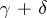for. Since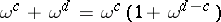, it suffices to determine the discrete logarithms for sums involving. This motivates the definition of the so-called Zech logarithm(which is actually due to C.G.J. Jacobi); thus,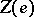is determined from the equation. Using discrete logarithms in conjunction with Zech logarithms is a useful representation in practical applications where repeated computations over a comparatively small finite field are required (with applications in coding theory being typical examples), since then the Zech logarithms can be pre-computed and, when needed for addition, retrieved by a simple table lookup. It is clear that a table lookup of Zech logarithms becomes impractical for large Galois fields. Thus the possibility of using this type of representation for large fields depends on the practicality of actually computing discrete logarithms, which is generally believed to be a very difficult problem. In fact, some systems in public-key cryptography (see Cryptography) are based on the intractability of computing discrete logarithms in sufficiently large Galois fields or, for state-of-the-art systems, in elliptic curves over Galois fields (cf. also Elliptic curve); see, e.g., [a5], [a13], [a16], [a17].

As of 2001, the standard reference on Galois fields is [a14]. In recent years there has been a resurgence of research in finite fields due to the wide variety of applications of various theoretical aspects of finite fields, e.g. in Galois geometry, coding theory (cf. Coding and decoding), design theory (cf. Block design; Difference set; Symmetric design), cryptography (cf. Cryptography; Cryptology), and signal processing. These applications usually require the use of efficient arithmetics, often in very large Galois fields; e.g., both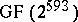andhave been used in commercial cryptographical devices. This has been one of the major motivations for studying the structural properties of proper Galois fields as sketched above in more detail. The interplay of structural and arithmetical properties is discussed in detail in [a10] and [a12]; computational and algorithmic aspects are treated in [a20]. Some good references for actual applications of Galois fields in the areas mentioned above are [a1], [a2], [a3], [a5], [a6], [a10], [a9], [a8], [a11], [a13], [a21], [a18], [a16], [a17], [a19]. A good reference for computational aspects is [a22].

How to Cite This Entry:
Galois field structure. Encyclopedia of Mathematics. URL: http://encyclopediaofmath.org/index.php?title=Galois_field_structure&oldid=15958
This article was adapted from an original article by Dieter Jungnickel (originator), which appeared in Encyclopedia of Mathematics - ISBN 1402006098. See original article